Know more about the Repeaters Batch Know more about the Repeaters Batch

# JEE Advanced Question Paper 2021 Physics Paper 1

On this page, the solutions for JEE Advanced Question Paper 2021 Physics Paper 1 are provided. The answers are explained in detail, with all the important steps included. Candidates can also access JEE Advanced 2021 Question paper and solutions in PDF format from this page. The JEE Advanced 2021 was held on October 3rd, 2021. The JEE Advanced question paper includes paper 1 and paper 2. It is mandatory for the candidates to attempt both papers. The question paper for the JEE Advanced 2021 Paper 1 Physics section is given below.

### JEE Advanced 2021 Paper 1 Physics Question Paper

Question 1: The smallest division on the main scale of Vernier calipers is 0.1 cm. Ten divisions of the Vernier scale correspond to nine divisions of the main scale. The figure below on the left shows the reading of this caliper with no gap between its two jaws. The figure on the right shows the reading with a solid sphere held between the jaws. The correct diameter of the sphere is;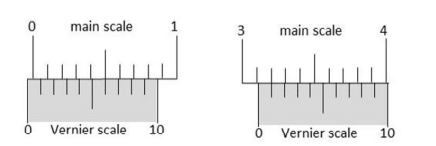1. a. 3.07 cm
2. b. 3.11 cm
3. c. 3.15 cm
4. d. 3.17 cm

Solution:

Least count of Vernier calipers = 0.01 cm

Error in scale = 4 LC = 0.04 cm

Reading = 3.1 cm + 1 L.C = 3.1 cm + 0.01 = 3.11 cm

So correct diameter of the sphere = (3.11 + 0.04) cm = 3.15 cm

Question 2: An ideal gas undergoes a four step cycle as shown in the P – V diagram below. During this cycle, heat is absorbed by the gas in;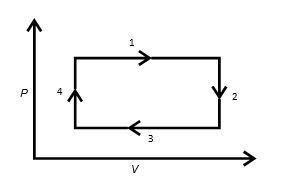1. a. steps 1 and 2
2. b. steps 1 and 3
3. c. steps 1 and 4
4. d. steps 2 and 4

Solution: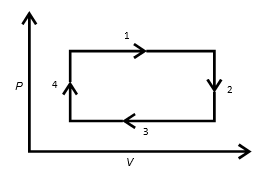Given P – V diagram

For process (1)

ΔQ1 = nCPΔT

As P = constant and V increases so T will increase

So △Q1 > 0

For process (2)

ΔQ2 = nCVΔT

V = constant, P↓, So T↓

For process (3), △Q3 = nCP△T < 0

For process (4), △Q4 = nCP△T

As △T > 0

So △Q4 > 0

Question 3: An extended object is placed at point O, 10 cm in front of a convex lens L1 and a concave lens L2 is placed 10 cm behind it, as shown in the figure. The radii of curvature of all the curved surfaces in both the lenses are 20 cm. The refractive index of both the lenses is 1.5. The total magnification of this lens syStatement is;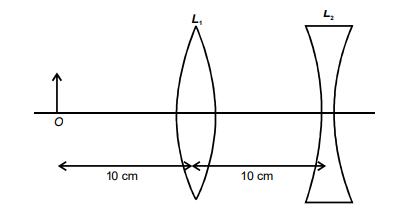1. a. 0.4
2. b. 0.8
3. c. 1.3
4. d. 1.6

Solution:

$$\frac{1}{f_{1}}= \left ( \frac{3}{2}-1 \right )(\frac{2}{20})$$
= 1/20

$$\frac{1}{v_{1}}-\frac{1}{-10}=\frac{1}{20}$$

$$\frac{1}{v_{1}}=\frac{1}{20}-\frac{1}{10}=\frac{-1}{20}$$

v1 = -20 cm

m1 = v/u1 = -20/-10 = 2

Again,

$$\frac{1}{v_{2}}-\frac{1}{-30}=\frac{1}{-20}$$

$$\frac{1}{v_{2}}=-\frac{1}{30}-\frac{1}{20}=-\frac{5}{50}=-\frac{1}{12}$$

m2 = -12/-30 = ⅖

m = m1 = m2 = 2 x (⅖) = 0.8

Question 4: A heavy nucleus Q of half-life 20 minutes undergoes alpha-decay with a probability of 60% and beta-decay with a probability of 40%. Initially, the number of Q nuclei is 1000. The number of alpha-decays of Q in the first one hour is;

1. a. 50
2. b. 75
3. c. 350
4. d. 525

Solution:

t1/2 = 20 min

In 60 min, no. of half-life = 3

$$\Rightarrow N_{A}=\left [ 1000-1000/2^{3} \right ]\times 0.6$$

= 100 x (⅞) x 0.6

= 525

Question Statement for Question Nos. 5 and 6

A projectile is thrown from a point O on the ground at an angle 45° from the vertical and with a speed of 5 √2 m/s. The projectile at the highest point of its trajectory splits into two equal parts. One part falls vertically down to the ground, 0.5 s after the splitting. The other part, t seconds after the splitting, falls to the ground at a distance x meters from the point O. The acceleration due to gravity g = 10 m/s2.

Question 5: The value of t is ________.

Solution:

H = u2sin2θ/2g

$$= \frac{50}{2\times 10}\times \frac{1}{2}=\frac{5}{4}$$

$$t= \sqrt{\frac{2H}{g}}= \sqrt{\frac{2\times 5}{4\times 10}}= (1/2) s = 0.5 s$$

Question 6: The value of x is ________.

Solution:

X = 3R/2 as Xcm = R

R = u2sin2θ/g

= 50/10 = 5

⇒X = 3R/2 = 15/2 = 7.5 m

Question Statement for Question Nos. 7 and 8

In the circuit shown below, the switch S is connected to position P for a long time so that the charge on the capacitor becomes q1 µC. Then S is switched to position Q. After a long time, the charge on the capacitor is q2 µC.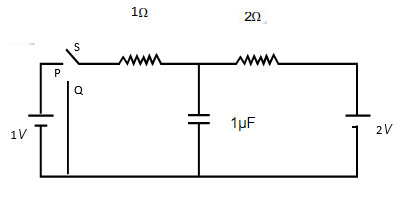Question 7: The magnitude of q1 is

Solution:

With switch S at position P after long time potential difference across capacitor branch

$$\frac{\frac{2}{2}+\frac{1}{1}}{\frac{1}{2}+\frac{1}{1}}=\frac{2\times 1}{3}=(4/3) v$$

Charge on capacitor q1μC = (4/3) μC

⇒q1 = 4/3 = 1.33

Question 8: The magnitude of q2 is_____.

Solution:

With switch S at position Q after long time potential difference across the capacitor

= potential difference across a resistance of 1 ohm.

= (⅔) v

Charge on capacitor q2μC = (2/3) μC

⇒ q2 = 0.67

Question Statement for Question Nos. 9 and 10

Two-point charges –Q and +Q / √3 are placed in the xy-plane at the origin (0, 0) and a point (2, 0), respectively, as shown in the figure. This results in an equipotential circle of radius R and potential V = 0 in the xy-plane with its centre at (b, 0). All lengths are measured in meters.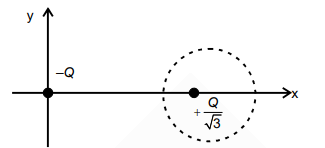Question 9:The value of R is ___ meter

Solution:

Question 10: The value of b is ___ meter

Solution:

Solution for Q. Nos. 9 & 10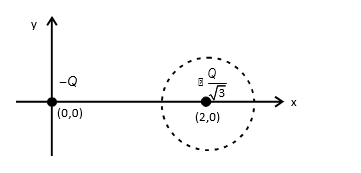$$V(x,y)=\frac{1}{4\pi \epsilon _{0}}\left [ -\frac{Q}{\sqrt{x^{2}+y^{2}}} +\frac{Q}{\sqrt{3}\sqrt{(x-2)^{2}+y^{2}}}\right ]$$

⇒ 3(x-1)2 + 3y2 =x2+y2

⇒ (x-3)2 + y2 = (√3)2

Question 11: A horizontal force F is applied at the centre of mass of a cylindrical object of mass m and radius R, perpendicular to its axis as shown in the figure. The coefficient of friction between the object and the ground is. The centre of mass of the object has an acceleration a. The acceleration due to gravity is g. Given that the object rolls without slipping, which of the following statement(s) is(are) correct?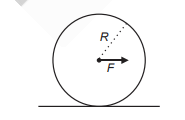1. a. For the same F, the value of a does not depend on whether the cylinder is solid or hollow
2. b. For a solid cylinder, the maximum possible value of a is 2g
3. c. The magnitude of the frictional force on the object due to the ground is always mg
4. d. For a thin-walled hollow cylinder, a = F/2m

Solution: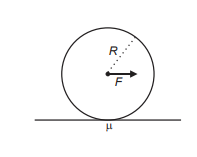For a solid cylinder,

$$F\times R= \frac{3}{2}mR^{2}\times \frac{a}{R}$$

⇒ a = 2F/3m

For hollow cylinder,

$$F\times R= 2mR^{2}\times \frac{a}{R}$$

⇒ a = F/3m

For solid cylinder

f = F - m x (2F/3m) = (F/3) ≤ μmg

⇒ F≤ 3μmg

Therefore,

A≤ (2/3m) x (3μmg)

⇒ amax = 2μg

Question 12: A wide slab consisting of two media of refractive indices n1 and n2 is placed in the air as shown in the figure. A ray of light is incident from medium n1 to n2 at an angle, where sin is slightly larger than 1/n1. Take the refractive index of air as 1. Which of the following statement(s) is(are) correct?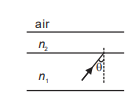1. a. The light ray enters air if n2 = n1
2. b. The light ray is finally reflected back into the medium of refractive index n1 if n2 < n1
3. c. The light ray is finally reflected back into the medium of refractive index n1 if n2 > n1
4. d. The light ray is reflected back into the medium of refractive index n1 if n2 = 1

Solution:

Sin θ ＞1/ n1 ... (i)

And n1 sinθ = 1 × sinr ... (ii)

⇒ sinr > 1

⇒ refraction into air is not possible

Question 13: A particle of mass M = 0.2 kg is initially at rest in the xy-plane at a point (x = –l, y = –h), where l = 10 m and h = 1 m. The particle is accelerated at time t = 0 with a constant acceleration a = 10 m/s2 along the positive x-direction. Its angular momentum and torque with respect to the origin, in SI units, are represented by

$$\vec{L}$$
and
$$\vec{\tau }$$
, respectively.
$$\hat{i},\hat{j},\hat{k}$$
, and are unit vectors along the positive x, y and z-directions respectively. If
$$\hat{k}=\hat{i}\times \hat{j}$$
then which of the following statement(s) is(are) correct?

1. a. The particle arrives at the point (x = l, y = –h) at time t = 2s
2. b.
$$\vec{\tau }=2\hat{k}$$
when the particle passes through the point (x = l, y = –h)
3. c.
$$\vec{L }=4\hat{k}$$
when the particle passes through the point (x = l, y = –h)
4. d.
$$\vec{\tau }=\hat{k}$$
when the particle passes through the point (x = 0, y = –h)

Solution: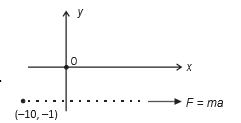$$t=\sqrt{\frac{2\times 20}{10}}=2 s$$

$$\vec{\tau }=(0.2 \times 10 \times 1)\hat{k}= 2\hat{k}$$

$$\vec{L}=(0.2 \times (10 \times2)\times 1)\hat{k}= 4\hat{k}$$

Question 14: Which of the following statement(s) is(are) correct about the spectrum of hydrogen atom?

1. a. The ratio of the longest wavelength to the shortest wavelength in the Balmer series is 9/5
2. b. There is an overlap between the wavelength ranges of Balmer and Paschen series
3. c. The wavelengths of Lyman series are given by (1 + 1/m20 where λ0 is the shortest wavelength of Lyman series and m is an integer
4. d. The wavelength ranges of the Lyman and Balmer series do not overlap

Solution:

For Balmer series:

$$\frac{1}{\lambda }= R\left [ \frac{1}{2^{2}}-\frac{1}{n^{2}} \right ]$$

n = 3,4,5 -----

$$\frac{1}{\lambda_{max} }= R\left [ \frac{1}{4}-\frac{1}{9} \right ]$$

$$\frac{1}{\lambda_{min} }= R\left [ \frac{1}{4}\right ]$$

$$\frac{\lambda _{max}}{\lambda _{min}}= \frac{9}{5}$$

For Layman Series

$$\frac{1}{\lambda }= R\left ( 1-\frac{1}{n^{2}} \right )$$

n = 2,3,4 ------

1/λmin = R

$$\Rightarrow \lambda =\lambda _{0}n^{2}/(n^{2}-1)$$

Question 15:A long straight wire carries a current, l = 2 ampere. A semi-circular conducting rod is placed beside it on two conducting parallel rails of negligible resistance. Both the rails are parallel to the wire. The wire, the rod and the rails lie in the same horizontal plane, as shown in the figure. Two ends of the semi-circular rod are at distances 1 cm and 4 cm from the wire. At time t = 0, the rod starts moving on the rails with a speed v = 3.0 m/s (see the figure).

A resistor R = 1.4 and a capacitor C0 = 5.0 F are connected in series between the rails. At time t = 0, C0 is uncharged. Which of the following statement(s) is(are) correct? [μ0 = 4 × 10–7 SI units. Take ln 2 = 0.7]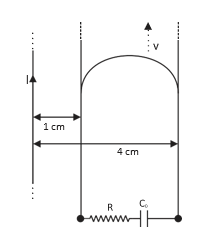1. a. Maximum current through R is 1.2 × 10–6 ampere
2. b. Maximum current through R is 3.8 × 10–6 ampere
3. c. Maximum charge on capacitor C0 is 8.4 × 10–12 coulomb
4. d. Maximum charge on capacitor C0 is 2.4 × 10–12 coulomb

Solution:

The equivalent circuit of the given arrangement is,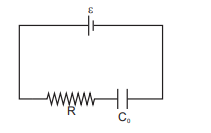$$\epsilon = \frac{\mu _{0}Iv}{2\pi }In\frac{b}{a}$$

= 1.68 × 10–6 V

At t = 0, imax = ε/R = 1.68 x 10-6/1.4

= 1.2 x 10-6 A

At t = ∞, qmax = C0ε = 8.4 x 10-12 C

Question 16: A cylindrical tube, with its base as shown in the figure, is filled with water. It is moving down with constant acceleration along a fixed inclined plane with an angle = 45º. P1 and P2 are pressures at points 1 and 2, respectively, located at the base of the tube. Let

$$\beta = P_{1}-P_{2}/\rho gd$$
, where ρ is the density of water, d is the inner diameter of the tube and g is the acceleration due to gravity. Which of the following statement(s) is(are) correct?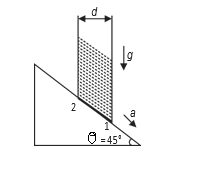1. a. β = 0 when a = g/√2
2. b. β ＞0 when a = g/√2
3. c.
$$\beta = \frac{\sqrt{2}-1}{\sqrt{2}}$$
when a = g/2
4. d. β = 1/√2 when a = g/√2

Solution: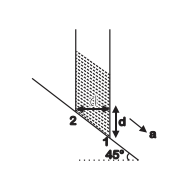P1 = P2 – acos45°d + ρ(g – asin45°)d

⇒P1-P2/ρgd = 1 - √2a/g

⇒ β = 0 for a = g/√2

$$\beta = \frac{\sqrt{2}-1}{\sqrt{2}}$$
when a = g/2

Question 17: An α -particle (mass 4 amu) and a singly charged sulfur ion (mass 32 amu) are initially at rest. They are accelerated through a potential V and then allowed to pass into a region of a uniform magnetic field which is normal to the velocities of the particles. Within this region, the -particle and the sulfur ion move in circular orbits of radii rα and rs respectively. The ratio rs/rα is ________.

Solution:

r = mv0/qB

(½)mv02 = qV

$$r = \sqrt{2mqV}/qB$$

$$r = \frac{1}{B}\sqrt{\frac{2mV}{q}}$$

$$\frac{r_{s}}{r_{\alpha } }=\sqrt{\frac{m_{s}}{q_{s}}}\times\sqrt{\frac{q_{\alpha }}{m_{\alpha }}} =\sqrt{2}\times \sqrt{8}$$

$$\frac{r_{s}}{r_{\alpha } }= 4$$

Question 18: A thin rod of mass M and length a is free to rotate in a horizontal plane about a fixed vertical axis passing through point O. A thin circular disc of mass M and of radius a/4 is pivoted on this rod with its centre at a distance a/4 from the free end so that it can rotate freely about its vertical axis, as shown in the figure. Assume that both the rod and the disc have uniform density and they remain horizontal during the motion. An outside stationary observer finds the rod rotating with an angular velocity and the disc rotating about its vertical axis with angular velocity 4Ω. The total angular momentum of the syStatement about the point O is

$$\left (\frac{Ma^{2}\Omega }{48}\right )n$$
. The value of n is _________.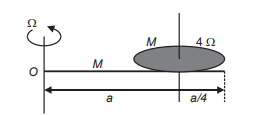Solution:

Ls= Ldisc + Lrod

$$L_{disc}= \vec{r}\times \vec{p}+I_{cm}4\Omega$$

$$= \frac{Ma^{2}}{32}\times 4\Omega +\frac{3a}{4}\times \frac{3a}{4}\times M\Omega$$

= (11/16) Ma2 Ω

Lrod = Ma2 Ω/3

$$L_{syStatement}= \frac{Ma^{2}}{3}\Omega +\frac{11}{16}Ma^{2}\Omega$$

= (49/48) Ma2Ω

n = 49

Question 19: A small object is placed at the centre of a large evacuated hollow spherical container. Assume that the container is maintained at 0 K. At time t = 0, the temperature of the object is 200 K. The temperature of the object becomes 100 K at t = t1 and 50 K at t = t 2 . Assume the object and the container to be ideal black bodies. The heat capacity of the object does not depend on temperature. The ratio (t1/t2) is ______.

Solution:

= KT4

-mS(dT/dt) = KT4

$$-mS\int_{200}^{100}\frac{dT}{T^{4}}=Kt_{1}$$

$$t_{1}=\frac{1}{K_{1}}\left [ \frac{1}{100^{3}}-\frac{1}{200^{3}} \right ]=\frac{1}{K_{1}}\left [ \frac{7}{200^{3}} \right ]$$

$$t_{2}=\frac{1}{K_{1}}\left [ \frac{1}{50^{3}}-\frac{1}{200^{3}} \right ]=\frac{1}{K_{1}}\left [ \frac{63}{200^{3}} \right ]$$

$$\frac{t_{2}}{t_{1}}=9$$

### JEE Advanced 2021 Physics Paper 1 Solutions